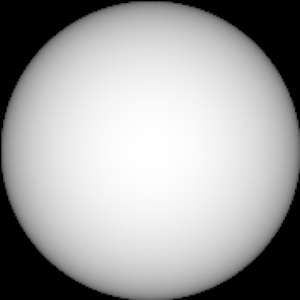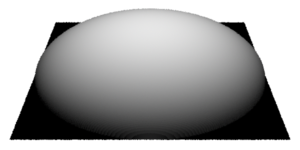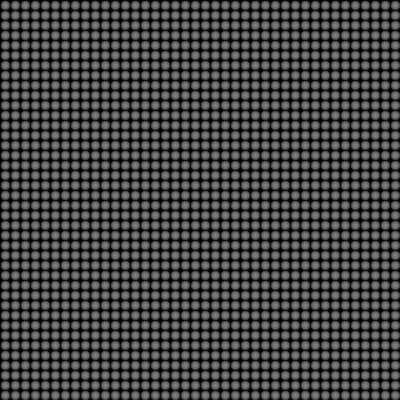### RSLBumps from Lights

#### Introduction

This tutorial presents a technique for generating a 32 bit image that is suitable, once converted to a texture, for use as a displacement map that will produce a pattern of high frequency bumps. The 32 bit image is derived from an orthographic rendering of a square polygon lit by instances of a custom light source shader. The shader is implemented such that its lighting distribution, figure 1, produces a gradation of grayscale values on the square polygon that will cause the displacement map to produce hemi-spherical "bumps".Figure 1
Light source - spherical drop-off profile

For example, the image shown in figure 2 was converted to a texture and used as a map to displace the polygon shown in figure 3.Figure 2
Orthographic image of a polygon
illuminated by a single custom light source.Figure 3
A polygon displaced by a texture map
from orthographic rendering of figure 2.

The tutorial uses a python script to write a pre-baked (archive) rib file that references an arbitary number of light sources. For example, figure 3 is an image of a polygon lit by 1600 lights distributed in a regular grid. Figure 4 shows the effect of using the image as a displacement map on a nurbs sphere. Despite the very high frequency of the bumps at the pole of the sphere the pattern displays very little aliasing - a benefit derived from the use of a texture map.Figure 3Figure 4

#### Basic Code

Because the bumps for the final image were required to be hemi-spherical, a custom light source shader, called `BumpPointLight`, was implemented. Its source code is shown in the first listing. The fall-off of the light has a profile shown in figure 1.

Listing 1 (bumpPointLight.sl)

 ```light bumpPointLight(float intensity = 1, radius = 0.01; string __category = "bumpLight"; output varying float _output = 0; ) { illuminate(point "shader"(0,0,0)) { float dist = length(L); if(dist >= radius) _output = 0; else _output = sqrt(radius * radius - dist * dist); _output = _output * intensity; Cl = _output; } }```

The light source shader uses an output variable of type float so that its value can be used directly by the surface shader shown in listing 2.

Listing 2 (bumpPointSurface.sl)

 ```surface bumpPointSurface(float Kb = 4) { float totalGray = 0, gray; illuminance("bumpLight", P) { if(lightsource("_output", gray)) { totalGray += gray; } } Oi = Os; Ci = Oi * Cs * totalGray * Kb; }```

Listing 3 is the python code that writes a pre-baked rib file that references the custom light source shader. If the script is run with Cutter the output rib file will be saved to the Cutter directory.

Listing 3 (regularGridOfLights.py)

 ```import sys, math, random def regularGridOfLights(fileID, rad): dia = rad * 2 repeats = 1/dia print("Lighting grid has %s by %s light sources" % (int(repeats),int(repeats))) count = 1 for x in range(int(repeats)): for z in range(int(repeats)): fileID.write('TransformBegin\n') fileID.write('\tTranslate %s %s %s\n' % (dia * (x + 0.5), 0, dia * (z + 0.5))) fileID.write('\tLightSource "bumpPointLight" %s "radius" %s\n' % (count, rad)) fileID.write('TransformEnd\n') count = count + 1 def main(): fileID = open('./bumpPointLight.rib','w') radius = 0.0125 regularGridOfLights(fileID, radius) fileID.close() print 'completed' if __name__ == "__main__": main() ```

Finally, listing 4 provides a rib file that uses the baked lighting rig produced by regularGridOfLights.py. The rib file is setup so that a 32 bit secondary image named "bumps.tif" is saved to the Cutter directory. The `system` call after `WorldEnd` converts the secondary image to a texture. The texture can subsequently be used to displace other objects.

Listing 4 (makeTexture.rib)

 ```DisplayChannel "color Ci" Display "bumps" "it" "rgba" Display "+./bumps.tif" "tiff" "Ci" Format 256 256 1 # <<-- use for initial tests #Format 4096 4096 1 # <<-- use for final tests Projection "orthographic" ShadingRate 1 ScreenWindow -0.5 0.5 -0.5 0.5 Translate 0 0 1 Rotate -90 1 0 0 Rotate 0 0 1 0 Scale 1 1 -1 WorldBegin TransformBegin Translate -0.5 0.0 -0.5 ReadArchive "./bumpPointLight.rib" TransformEnd Surface "BumpPointSurface" "Kb" 40 AttributeBegin Polygon "P" [-0.5 0 -0.5 -0.5 0 0.5 0.5 0 0.5 0.5 0 -0.5] "st" [0 0 0 1 1 1 1 0] AttributeEnd WorldEnd System "txmake -mode periodic ./bumps.tif ./bumps.tex" ```